Question

# Enter your answer in the provided box. A buffer that contains 1.10 M base, B, and 0.700 M of its conjugate acid, BH, ha...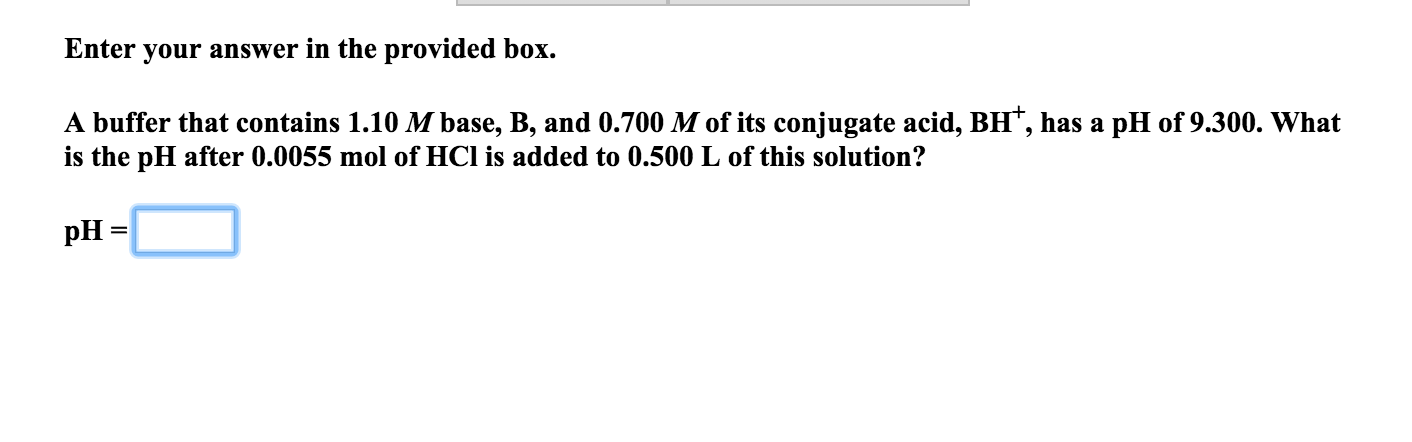Enter your answer in the provided box. A buffer that contains 1.10 M base, B, and 0.700 M of its conjugate acid, BH, has a pH of 9.300. What is the pH after 0.0055 mol of HCl is added to 0.500 L of this solution? pH =

pH = pKa + log [B / BH+]

9.300 = pKa + log (1.10 / 0.70 )

pKa = 9.10

molarity of HCl = 0.0055 / 0.50 = 0.011 M = C

pH = pKa + log [B - C / BH+ + C]

pH = 9.10 + log (1.10 -0.011 / 0.7 + 0.011)

pH = 9.28

#### Earn Coins

Coins can be redeemed for fabulous gifts.

Similar Homework Help Questions
• ### Enter your answer in the provided box. A buffer that contains 1.05 M base, B, and...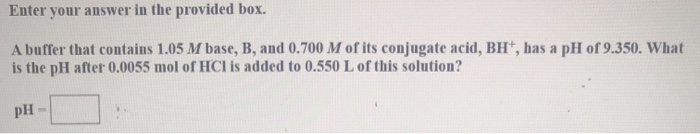Enter your answer in the provided box. A buffer that contains 1.05 M base, B, and 0.700 M of its conjugate acid, BH, has a pH of 9.350. What is the pH after: 0.0055 mol of HCl is added to 0.550 L of this solution? pH

• ### Enter your answer in the provided box. A buffer that contains 1.00 M base, B, and...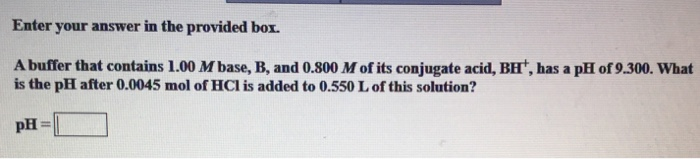Enter your answer in the provided box. A buffer that contains 1.00 M base, B, and 0.800 M of its conjugate acid, BH', has a pH of 9.300. What is the pH after 0.0045 mol of HCl is added to 0.550 L of this solution? pH =

• ### Enter your answer in the provided box. A buffer that contains 0.368 M base, B, and 0.229 M of its conjugate acid, BH...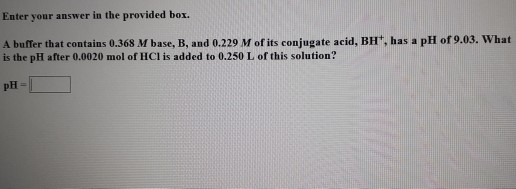Enter your answer in the provided box. A buffer that contains 0.368 M base, B, and 0.229 M of its conjugate acid, BH, has a pH of 9.03. What is the pH after 0.0020 mol of HCl is added to 0.250 L of this solution?

• ### Enter your answer in the provided box. A buffer that contains 0.388 M base, B, and 0.283 M of its conjugate acid, BH+,...

Enter your answer in the provided box. A buffer that contains 0.388 M base, B, and 0.283 M of its conjugate acid, BH+, has a pH of 8.99. What is the pH after 0.0020 mol of HCl is added to 0.250 L of this solution? pH =

• ### Enter your answer in the provided box. A buffer that contains 1.00 M base, B, and...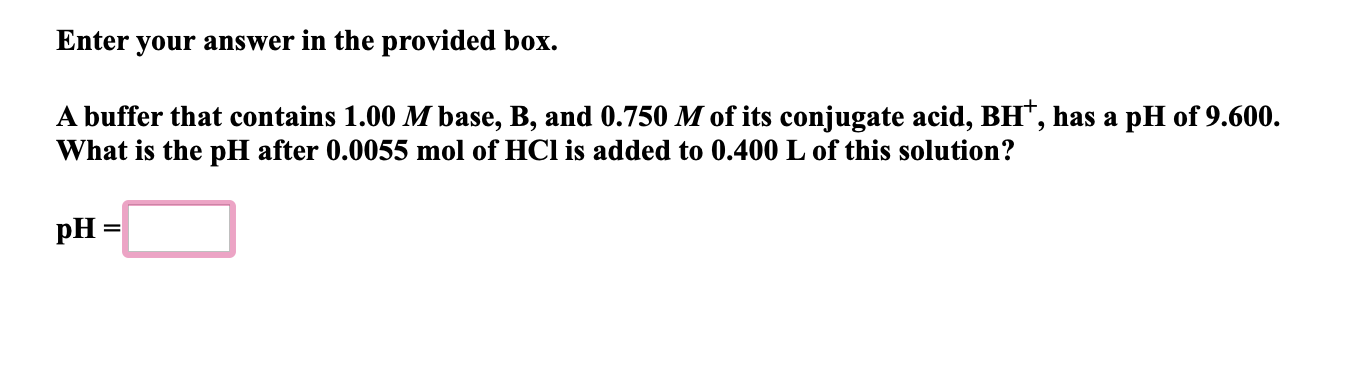Enter your answer in the provided box. A buffer that contains 1.00 M base, B, and 0.750 M of its conjugate acid, BH, has a pH of 9.600. What is the pH after 0.0055 mol of HCl is added to 0.400 L of this solution? pH =

• ### I was not given the Kb. Enter your answer in the provided box. A buffer that...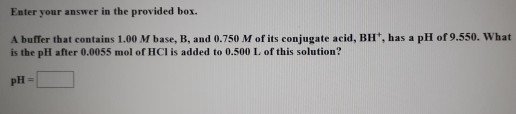I was not given the Kb. Enter your answer in the provided box. A buffer that contains 1.00 M base, B, and 0.750 M of its conjugate acid, BH, has a pH of 9.550. What is the pH after 0.0055 mol of HCl is added to 0.500 L of this solution? pH =

• ### Enter your answer in the provided box. A buffer that contains 0.459 M base, B, and...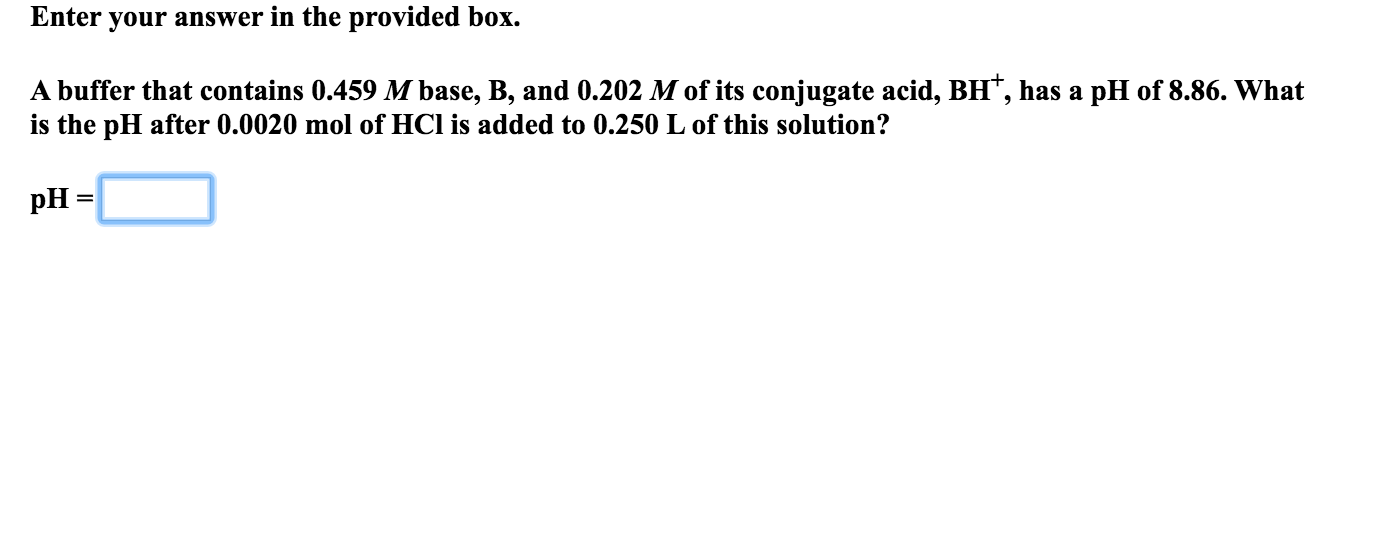Enter your answer in the provided box. A buffer that contains 0.459 M base, B, and 0.202 M of its conjugate acid, BH, has a pH of 8.86. What is the pH after 0.0020 mol of HCl is added to 0.250 L of this solution? pH =

• ### Enter your answer in the provided box. A buffer that contains 0.564 M base, B, and...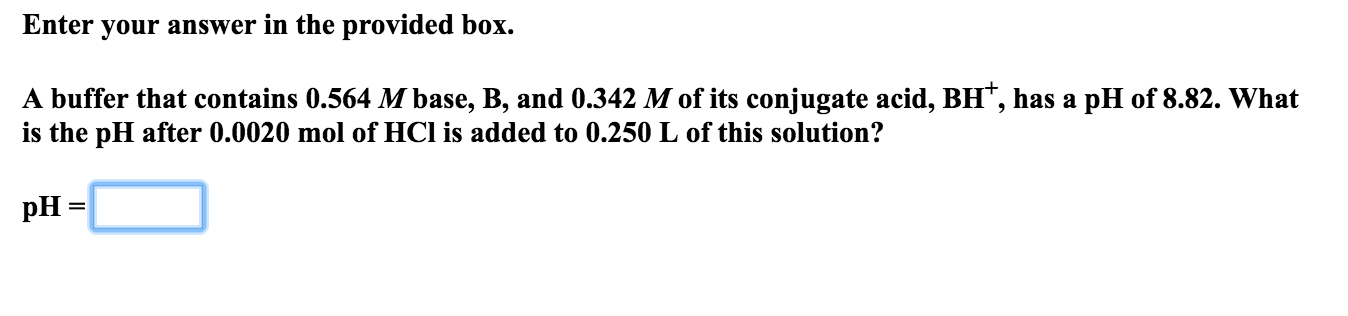Enter your answer in the provided box. A buffer that contains 0.564 M base, B, and 0.342 M of its conjugate acid, BH, has a pH of 8.82. What is the pH after 0.0020 mol of HCl is added to 0.250 L of this solution? pH =

• ### Enter your answer in the provided box. A buffer that contains 0.531 M base, B, and...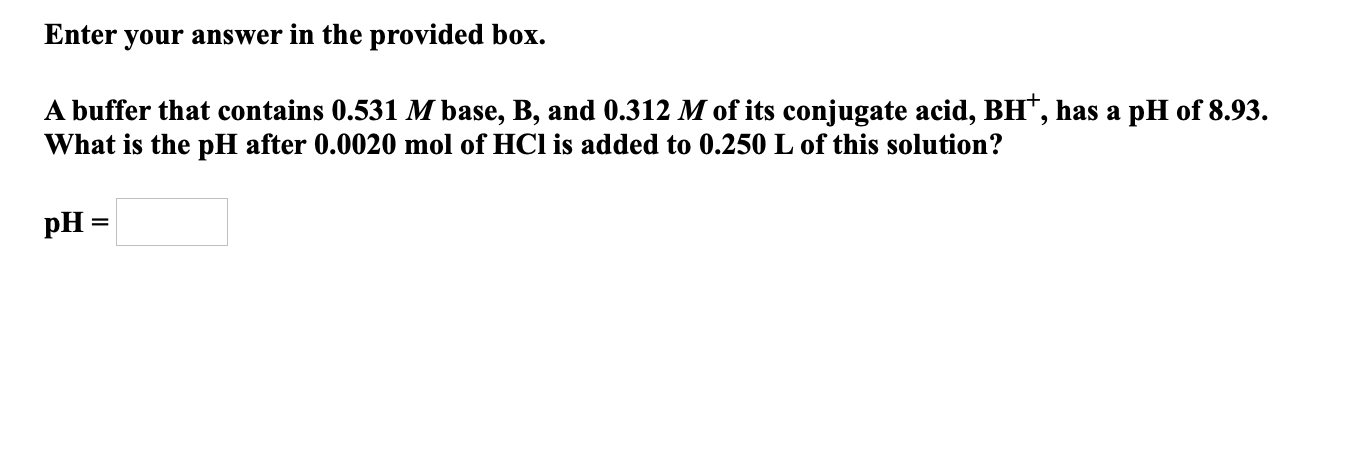Enter your answer in the provided box. A buffer that contains 0.531 M base, B, and 0.312 M of its conjugate acid, BH, has a pH of 8.93. What is the pH after 0.0020 mol of HCl is added to 0.250 L of this solution? pH=

• ### Enter your answer in the provided box. A buffer that contains 0.457 M base, B, and...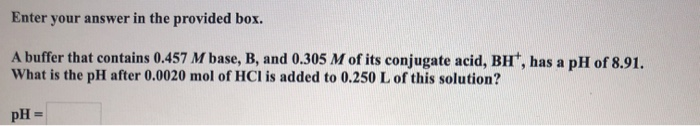Enter your answer in the provided box. A buffer that contains 0.457 M base, B, and 0.305 M of its conjugate acid, BH, has a pH of 8.91. What is the pH after 0.0020 mol of HCl is added to 0.250 L of this solution? pH =